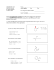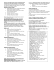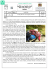# Blank PDF

## Transcription

Blank PDF
```EF 152 Exam #1, Fall, 2014
Page 1 of 6
Name:
Section: ________
Instructions
• Do not open the exam until instructed to do so.
• Do not leave if there is less than 5 minutes to go in the exam.
• When time is called, immediately stop writing, remain seated, and pass your exam to the center aisle.
• Do not stand up or leave until all exams have been collected.
• Working after time is called results in an automatic 10 point deduction.
Guidelines
• Assume 3 significant figures for all given numbers unless otherwise stated
• Show all of your work – no work, no credit
Stress and Strain
Stress =
Strain =
=
=
Bernoulli’s Equation
\$
&
∆
Lift
≅ '-+(
!
WX
Density of Water
3
62.4 lb/ft
3
1000 kg/m
Atmospheric Pressure
101.3 kPa
14.7 psi
Conversions
3
1 ft = 7.48 gal
3
1 m = 1000 L
Uniform Circular Motion
Mass density
2
3
Weight density
5
3
= 6789(
Poiseuille’s Equation
uv =
DE
9b
gb
= 1.0 × 10 Pa ∙ s
AK
wB
<
= 9t &
Specific gravity
(relative density)
m
`=
;< = ([email protected] A?B )
( = t9
mnopqr
or
U B
s
snopqr
NY
P [\
(]^_ = Z
Stokes’ Law
8F
STB
N∙YP
Fluids
p – pressure
h – height
ρ – mass density
v – velocity
K – empirical constant
A – area
d – depth
"# – pressure on top of fluid
:=
=
(=Z
<
= ')1
!
<B
Satellites
Buoyant force
0
<B
2P
Kepler’s Third Law
VB
(&;)B
&
" = '). + "#
=
[email protected] 2B
O = 6.674 × 10A\$\$
Pressure in a fluid
Factor of Safety (FS)
=O
)=O
(\$ +\$ = (& +&
=
4=
N
&
Continuity
∆ =
'=
Universal Law of Gravitation
\$
"\$ + '(\$& + ')ℎ\$ = "& + '(&& + ')ℎ&
&N∙YP
<P
&;
a
= 6.38 × 10e m
= 5.97 × 10&j kg
Ellipse
eccentricity:
x=
&W
semi-major axis:
u=
(any curve)
Constant acceleration
\$
~ = ~# + (# • + u• &
&
( = (# + u•
∆~ =
<yz{ A<y|}
w B Aw€B
&W
<yz{ [<y|}
&
EF 152 Exam #1, Fall, 2014
Page 2 of 6
EF 152 Exam #1, Fall, 2014
Page 3 of 6
1. (2 pts) The volume of a 10 lb bowling ball is 0.186 ft3. Will this bowling ball sink or float in water?
a. sink
b. float
2. (2 pts) Object A weighs 50 lb. Object B weighs 25 lb. How do their respective escape velocities compare?
a. vA = ¼ vB
b. vA = ½ vB
c. vA = vB
d. vA = 2 vB
e. vA = 4 vB
3. (2 pts) In a Venturi tube, how does the pressure in the restriction (p2)
compare to the inlet pressure (p1)?
a. p2 > p1
b. p2 = p1
c. p2 < p1
4. (8 pts) A satellite orbits the earth such that its period is 18 hours (64800 sec). Determine the height of the
satellite above the earth’s surface.
EF 152 Exam #1, Fall, 2014
Page 4 of 6
5. (6 pts) Determine the cross-sectional area of a 20 ft long, steel column that shortens 0.03 inches under a
5000 lb load. Esteel = 29x106 psi.
6. (16 pts) An L-bracket is supported by a pin at A and a cable at B as shown.
Determine the x-component of the reaction force at A.
A separate, complete FBD is required for full credit.
y
cable
50°
x
B
4 ft
16 lb
A
2 ft
3 ft
EF 152 Exam #1, Fall, 2014
Page 5 of 6
7. (16 pts) Chris takes a break while pushing a 75 lb bookshelf up a ramp as shown. Determine the magnitude
of the force P required to keep the bookshelf from tipping down the ramp.
A separate, complete FBD is required for full credit.
CG
10°
P
25°
8. (16 pts) An 8 ft x 8 ft x 2 ft floating dock weighs 3500 lb and is anchored to the bottom of a lake by a steel
cable with a cross-sectional area of 0.15 in2 and an ultimate strength of 270x103 psi. Due to flooding, the
dock is completely submerged. Determine the factor of safety of the cable.
A separate, complete FBD is required for full credit.
water level
DOCK
cable
lake bottom
EF 152 Exam #1, Fall, 2014
Page 6 of 6
9. (16 pts) A warehouse is 5.5m tall and equipped with a fire sprinkler system. The system delivers water at a
rate of 0.02 m3/s. The main pipe leaving the pump has an area of 6.4x10-3 m2 and the pipe delivering water
to the sprinklers has an area of 1.2x10-3 m2. Determine the pressure provided by the pump such that water
is delivered to the sprinklers at 350 kPa.
to sprinklers
Pump
10. (16 pts) A glass marble (radius = 0.015m) falling through olive oil reaches a terminal velocity of 4.56 m/s.
j
Determine the mass density of olive oil. Vsphere = 79 K ;
FBD required for full credit
K
'glass = 2200 kg/m3 ; 8 olive oil = 0.138 Pa·s
```

### Problem Set 3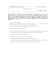### Exam 3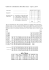### 10.1\\\-10.3 review ans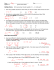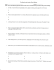### homework 4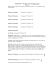### Automata Theory Sample Midterm Exam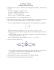### Math 0120 Examination #3 Sample Name (Print)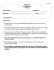### Math 30G – Calculus – Sample Final Exam Fall 2012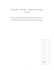### Assignment 2 the 1.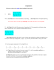### 3w 3 t5+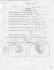### Exam 3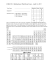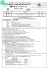### 1110 Chocolate Science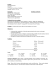### Econ 230B Spring 2015 FINAL EXAM: Solutions The average grade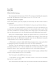### Äá» THI THá»¬ VÃO Lá»P 10 CHUYÃN THPT NÄm há»c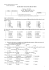### Safety of Machinery and Control Systems - Directive for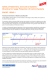### CHEMISTRY 3331 - Olafs Daugulis